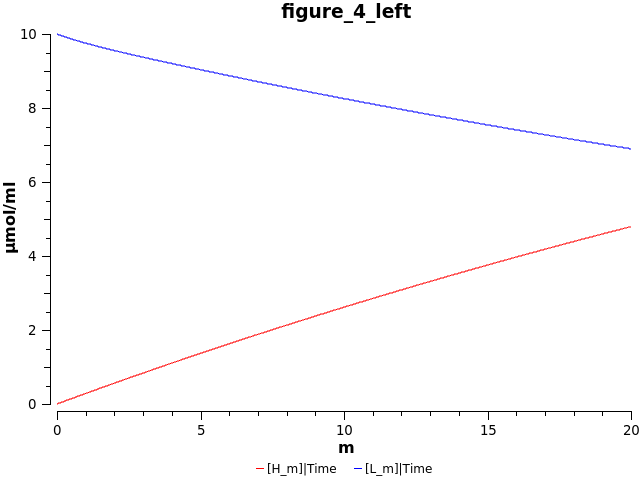## Evans2004 - Cell based mathematical model of topotecanModel Identifier
BIOMD0000000945
Short description

A two compartment mathematical model of the antineoplastic compound topotecan

Format
SBML (L2V4)
Related Publication
• A mathematical model for the in vitro kinetics of the anti-cancer agent topotecan.• Evans ND, Errington RJ, Shelley M, Feeney GP, Chapman MJ, Godfrey KR, Smith PJ, Chappell MJ
• Mathematical biosciences , 6/ 2004 , Volume 189 , Issue 2 , pages: 185-217 , PubMed ID: 15094319
• School of Engineering, University of Warwick, Coventry CV4 7AL, UK.
• In this paper a compartmental modelling approach is applied to provide a mathematical description of the activity of the anti-cancer agent topotecan, and delivery to its nuclear DNA target following administration. The activity of topotecan in defined buffers is first modelled using a linear two compartment model that then forms the basis of a cell based model for drug activity in live cell experiments. An identifiability analysis is performed before parameter estimation to ensure that the model output (i.e., continuous, perfect and noise-free data) uniquely determines the parameters. Parameter estimation is performed using experimental data which offers concentrations of active and inactive forms of topotecan from high performance liquid chromatography methods.
Contributors
Submitter of the first revision: Matthew Roberts
Submitter of this revision: Krishna Kumar Tiwari
Modellers: Matthew Roberts, Krishna Kumar Tiwari

is (2 statements)
BioModels Database MODEL1805170002
BioModels Database BIOMD0000000945

isDescribedBy (1 statement)
PubMed 15094319

hasTaxon (1 statement)
Taxonomy Homo sapiens

hasProperty (1 statement)
Mathematical Modelling Ontology Ordinary differential equation model

hasPart (2 statements)
isVersionOf (1 statement)
Pathway Ontology antineoplastic drug pathway

occursIn (1 statement)
Experimental Factor Ontology SU-DHL-4

Curation status
Curated

Modelling approach(es)

Tags

#### Connected external resources

SBGN view in Newt Editor

Name Description Size Actions

### Model files

Evans2004.xml SBML L2V4 representation of Evans2004 - Cell based mathematical model of topotecan 33.91 KB Preview | Download

Evans2004.cps Curated and annotated model COPASI file. 49.39 KB Preview | Download
• Model originally submitted by : Matthew Roberts
• Submitted: May 17, 2018 2:57:06 PM
##### Revisions

(*) You might be seeing discontinuous revisions as only public revisions are displayed here. Any private revisionsof this model will only be shown to the submitter and their collaborators.

Legends
: Variable used inside SBML models

Species
Reactions
Reactions Rate Parameters
L_c = (((k_i*v_1*L_m-(k_e+k_o_c)*L_c)+k_c_c*H_c)-k_b*(B_T-L_n)*L_c)+v_2*k_d*L_n (((k_i*v_1*L_m-(k_e+k_o_c)*L_c)+k_c_c*H_c)-k_b*(B_T-L_n)*L_c)+v_2*k_d*L_n k_c_c = 0.18637; k_i = 3.09E-4; k_b = 8.5341E-4; k_d = 4.4489; k_e = 1.014; v_2 = 0.393244873341375; v_1 = 6.0313630880579; B_T = 28.9; k_o_c = 0.026553
H_c = k_o_c*L_c-k_c_c*H_c k_o_c*L_c-k_c_c*H_c k_c_c = 0.18637; k_o_c = 0.026553
H_m = k_0_m*L_m-k_c_m*H_m k_0_m*L_m-k_c_m*H_m k_0_m = 0.0289; k_c_m = 1.06E-4
L_n = k_b*v_2*(B_T-L_n)*L_c-k_d*L_n k_b*v_2*(B_T-L_n)*L_c-k_d*L_n k_b = 8.5341E-4; k_d = 4.4489; v_2 = 0.393244873341375; B_T = 28.9
L_m = (-(k_0_m+k_i))*L_m+k_c_m*H_m+k_e*v_1*L_c (-(k_0_m+k_i))*L_m+k_c_m*H_m+k_e*v_1*L_c k_i = 3.09E-4; k_0_m = 0.0289; k_c_m = 1.06E-4; k_e = 1.014; v_1 = 6.0313630880579Curator's comment:
(added: 12 May 2020, 04:49:10, updated: 12 May 2020, 04:49:10)
Model is created using COPASI. Model parameter value v1 has been multiplied by 1e-3 in order produce something similar to figure 4 of the publication.